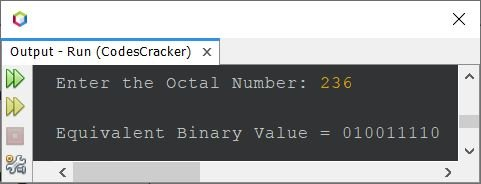# Java Program to Convert Octal to Binary

This article is created to cover a program in Java that converts an octal number entered by user at run-time of the program, to its equivalent binary value.

If you're not aware about, how the octal to binary conversion takes place, then refer to Octal to Binary. Now let's create the program.

## Octal to Binary in Java

The question is, write a Java program to convert octal number to binary. The octal value must be received by user at run-time. Answer to this question, is the program given below:

```import java.util.Scanner;

public class CodesCracker
{
public static void main(String[] args)
{
int i;
String binary="";

Scanner s = new Scanner(System.in);

System.out.print("Enter the Octal Number: ");
String octal = s.nextLine();

for(i=0; i<octal.length(); i++)
{
char ch = octal.charAt(i);
switch(ch)
{
case '0': binary = binary + "000";
break;
case '1': binary = binary + "001";
break;
case '2': binary = binary + "010";
break;
case '3': binary = binary + "011";
break;
case '4': binary = binary + "100";
break;
case '5': binary = binary + "101";
break;
case '6': binary = binary + "110";
break;
case '7': binary = binary + "111";
break;
default: System.out.println("\nInvalid Octal Digit!");
return;
}
}

System.out.println("\nEquivalent Binary Value = " +binary);
}
}```

The sample run of above program with user input 236 as octal number to convert and print its equivalent binary value, is shown in the snapshot given below:Here is another version of the program, that does the same job as of previous program. But internally, the code is different:

```import java.util.Scanner;

public class CodesCracker
{
public static void main(String[] args)
{
int octal, rev=0, rem;
String binary="";

Scanner s = new Scanner(System.in);

System.out.print("Enter the Octal Number: ");
octal = s.nextInt();

while(octal!=0)
{
rem = octal%10;
rev = (rev*10) + rem;
octal = octal/10;
}

octal = rev;

while(octal!=0)
{
rem = octal%10;
switch(rem)
{
case 0:
binary = binary + "000";
break;
case 1:
binary = binary + "001";
break;
case 2:
binary = binary + "010";
break;
case 3:
binary = binary + "011";
break;
case 4:
binary = binary + "100";
break;
case 5:
binary = binary + "101";
break;
case 6:
binary = binary + "110";
break;
case 7:
binary = binary + "111";
break;
default:
System.out.println("\nInvalid Octal Digit!");
return;
}
octal = octal/10;
}

System.out.println("\nEquivalent Binary Value = " +binary);
}
}```

#### Same Program in Other Languages

Java Online Test

« Previous Program Next Program »